### LOG10 Function Examples in Excel, VBA, & Google Sheets

This Tutorial demonstrates how to use the Excel LOG10 Function in Excel to calculate the base-10 logarithm of a number.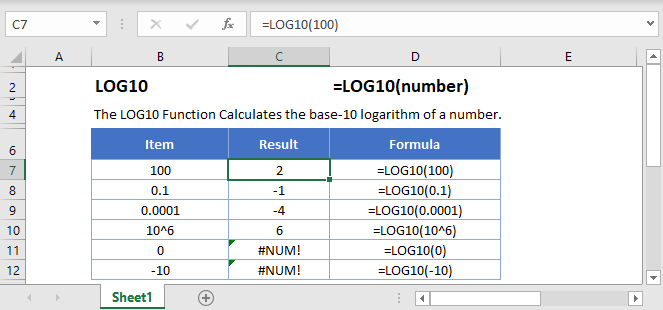## LOG10 Function Overview

The LOG10 Function Calculates the base-10 logarithm of a number.

To use the LOG10 Excel Worksheet Function, select a cell and type: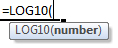(Notice how the formula inputs appear)

## LOG10 Function Syntax and Inputs:

number – A number.

AutoMacro - VBA Code Generator

## LOG10 Function

The LOG10 Function returns the logarithm of a number to base 10.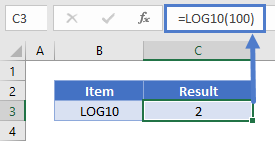## LOG10 Function – Decimal

The LOG10 Function can also return the logarithm of a decimal number to base 10.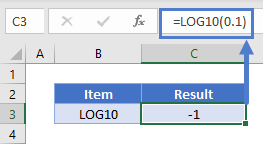## LOG10 Function – Negative Number/Zero

The LOG10 Function will return an error if the argument is zero or a negative number.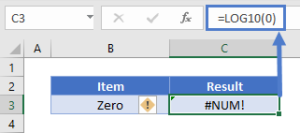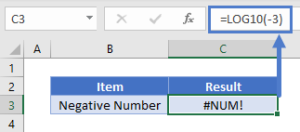## LOG10 Function – Power of 10

The LOG10 Function will return the exponent if the argument is expressed as a power of 10.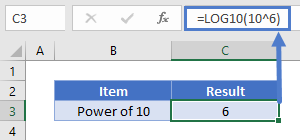## LOG10 in Google Sheets

The LOG10 Function works exactly the same in Google Sheets as in Excel: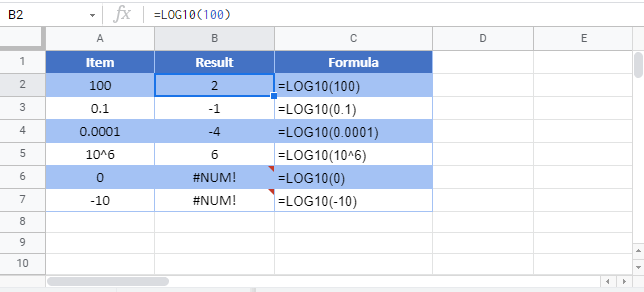## Additional Notes

Use the LOG10 Function to calculate the logarithm of a number to base-10. Simply enter any number directly into the formula, or reference a cell containing a number.

## LOG10 Examples in VBA

You can also use the LOG10 function in VBA. Type:
`application.worksheetfunction.log10(number)`

Executing the following VBA statements

will produce the following results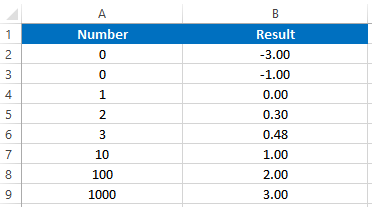For the function arguments (number, etc.), you can either enter them directly into the function, or define variables to use instead.

Return to the List of all Functions in Excel

## Excel Practice Worksheet

Practice Excel functions and formulas with our 100% free practice worksheets!

• Automatically Graded Exercises
• Learn Excel, Inside Excel!